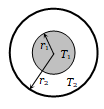• Subject:

...

• Topic:

...

The figure shows a system of two concentric spheres of radii ${\mathrm{r}}_{1}$ and ${\mathrm{r}}_{2}$ and kept at temperatures ${\mathrm{T}}_{1}$ and ${\mathrm{T}}_{2}$, respectively. The radial rate of flow of heat in a substance between the two concentric spheres is proportional to(a) $\frac{{\mathrm{r}}_{1}{\mathrm{r}}_{2}}{\left({\mathrm{r}}_{1}-{\mathrm{r}}_{2}\right)}$
(b) $\left({\mathrm{r}}_{1}-{\mathrm{r}}_{2}\right)$
(c) $\left({\mathrm{r}}_{1}-{\mathrm{r}}_{2}\right)\left({\mathrm{r}}_{1}{\mathrm{r}}_{2}\right)$
(d) In $\left(\frac{{\mathrm{r}}_{2}}{{\mathrm{r}}_{1}}\right)$